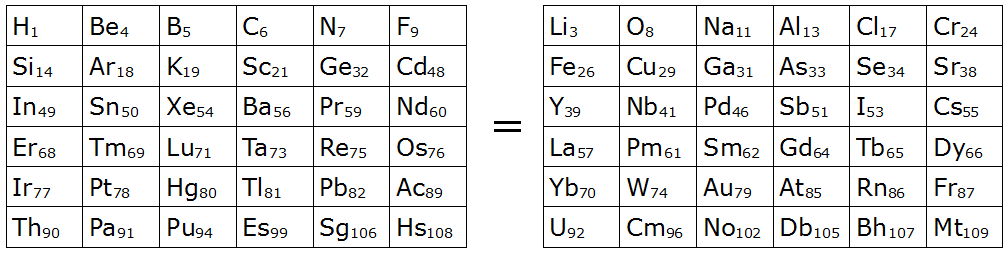# Alchemy

Mike Keith found this amazing correspondence in 2004. The two 6×6 squares below contain 72 different entries from the periodic table of the elements:The two squares are equal in three different ways:

1. If you spell out the name of each element listed (hydrogen, beryllium, etc.), the square on the left is an anagram of the square on the right.
2. The sum of the atomic numbers of the 36 elements on the left (2019) equals the sum of those on the right.
3. If you replace each symbol with its alphabetic score (where A=1, B=2, etc.; e.g. Li = L + I = 12 + 9 = 21), then the sum of the scores on the left (737) equals that of those on the right.

Keith writes, “The next largest pair of triply-equal squares like this would be 7×7 in size, containing a total of 98 different elements, [and] it seems quite unlikely that 98 of them could be so arranged. If this is true then the 6×6 pair presented here is the largest possible (at least for now, until many more new chemical elements have been discovered and named).”

(Mike Keith, “A Magical Pair of 6×6 Chemical Squares,” Word Ways, February 2004.)